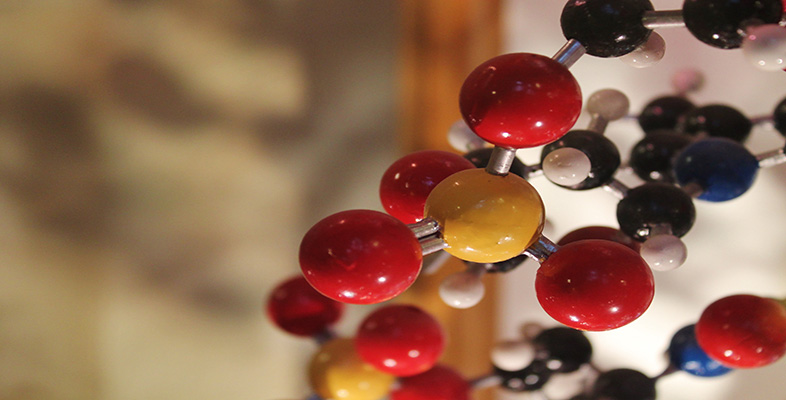Basic science: understanding numbers

Start this free course now. Just create an account and sign in. Enrol and complete the course for a free statement of participation or digital badge if available.

Free course

# 2.2 Calculating areas

Last week you discussed the SI unit for measuring length, the metre. In fact, you also discussed areas without paying too much attention to them – the area of the Earth, or of Alaska, for example. So, let’s talk more about areas and how to calculate them.

## Simple shapes

The areas of shapes like rectangles (and squares, which are a special type of rectangle) can be worked out by simply multiplying the two side lengths together. A rectangular room that is 3 metres long by 4 metres wide has an area of 3 multiplied by 4. The units for area are simply metres multiplied by metres, or as it is normally written, m2 (pronounced ‘metres squared’). So the area of the room is 12 m2, or twelve square metres.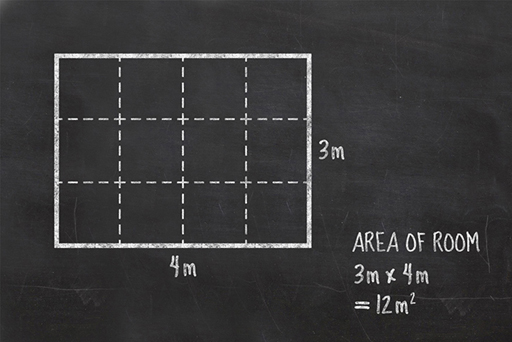Figure _unit2.3.1 Figure 1 Calculating the area of a rectangular room

Areas of squares and rectangles are fairly easy, but what about other shapes? It turns out that for a lot of simple shapes, there are some very simple methods that have already been shown to work. You can make a triangle by folding a square down the diagonal between two opposite corners. This square has an area of 4 m × 4 m = 16 m2 so each of the triangles is half that area or 8 m2.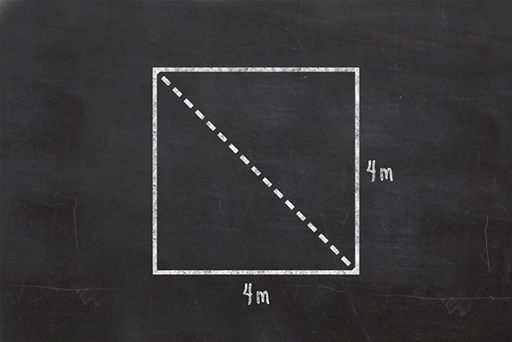Figure _unit2.3.2 Figure 2 Folding a square down the diagonal between two opposite corners

All triangles can be split by a line that makes the two parts either half of a square or rectangle, or one of each.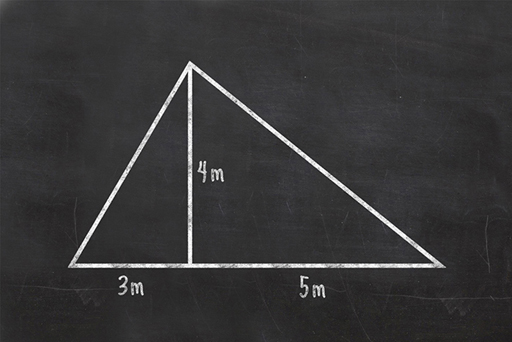Figure _unit2.3.3 Figure 3 A triangle that can’t be folded from a square or rectangle can be divided into smaller triangles that can

Since the area of each smaller triangle is easy to work out, being half of the ‘whole’ rectangle or square, the area of the larger triangle is also easy to work out, as it is just those two areas added together. If you are thinking ahead, you will have worked out that the end result of this is the same as multiplying the total base (3+5) by the height (4) and dividing that answer in half. So the area of a triangle is half of the base times the height.

Circles are a bit different but have their own method. The distance across a circle is called the diameter. Half of the diameter is the radius. The distance around the outside of a circle is called the circumference.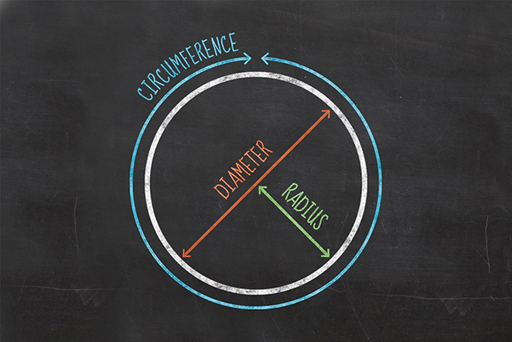Figure _unit2.3.4 Figure 4 The diameter, radius and circumference of a circle

The circumference of any circle is always 3.14 times its diameter … well roughly that. It is actually an unending string of numbers after the decimal point: 3.141592653589793238462643383279502884197169399375105820974944 … . It has so many numbers in it that we make life easier by giving it a name instead. We call it pi, which is a Greek letter that is often written as π.

The rule for working out the area of a circle is pi times the radius times the radius, or πr2. So, if the circle is 4 metres across (i.e. the radius is 2 metres), then the area is roughly 12.5 m2.

## Complex shapes

If you want to calculate the area of a country, you might think it is a horribly complicated process with all kinds of complex maths, but it can be done with a very simple, albeit long-winded method. All you need to do is divide the country into lots of small triangles and work out the total of all of their individual areas. Modern computing can do this in the blink of an eye from satellite images.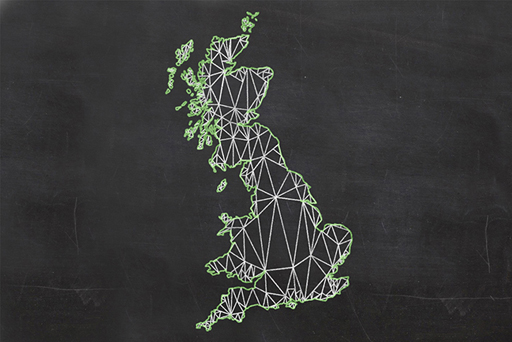Figure _unit2.3.5 Figure 5 Calculating the area of the United Kingdom by dividing it into triangles